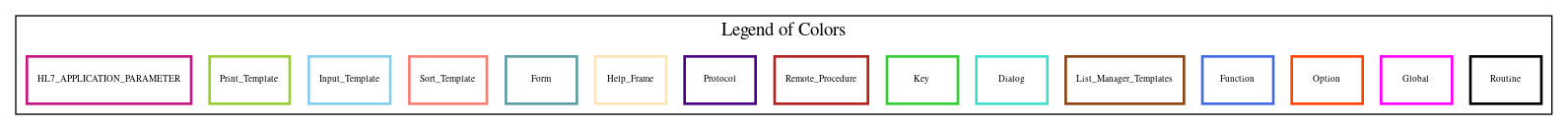Home   Package List   Routine Alphabetical List   Global Alphabetical List   FileMan Files List   FileMan Sub-Files List   Package Component Lists   Package-Namespace Mapping
Routine: SCCVCST4

# Routine: SCCVCST4

## Information

SCCVCST4 ;ALB/TMP - Scheduling Conversion Template Utilities - CST; APR 20, 1998

## Source Information

Source file <SCCVCST4.m>

## Call Graph### Call Graph Total: 9

Package Total Call Graph
Scheduling 6 (STOPS,ZERO)^SCCVEAE  EN^SCCVEAE1  ZERO^SCCVEAP  EN^SCCVEAP1  ZERO^SCCVEDI  EN^SCCVEDI1
VA FileMan 2 EN^DIQ  ^DIR
Kernel 1 \$\$FMTE^XLFDT

## Caller Graph

### Legends:#### Package Component Superscript legend

 action A extended action Ea event driver Ed subscriber Su protocol O limited protocol LP run routine RR broker B edit E server Se print P screenman SM inquire I### Caller Graph Total: 2

Package Total Caller Graph
Scheduling 2 SCCVCST3  SCCVCST5

## Entry Points

RESULT ; Display conversion results message
NOENT(SCCVTYPN,SCCVDFN,SCDTM) ;No entry was found for date/time/pt
DISPERR(SCERR,SCF) ; Display error
DISP1(SCCVTYPN,SCFILE1,SCCVDA) ; Display selected entry
; Conversion will include any child encounters
CONVQ
SCERR ; Invalid reasons

## External References

Name Field # of Occurrence
EN^DIQ DISP1+5
^DIR RESULT+9, NOENT+5, DISPERR+8, DISP1+8
STOPS^SCCVEAE CONV1+34
ZERO^SCCVEAE CONV1+41
EN^SCCVEAE1 CONV1+42
ZERO^SCCVEAP CONV1+17
EN^SCCVEAP1 CONV1+18
ZERO^SCCVEDI CONV1+25
EN^SCCVEDI1 CONV1+26
\$\$FMTE^XLFDT NOENT+5

## Interaction Calls

Name Line Occurrences
Function Call: WRITE
• Prompt: !
• Line Location: DISPERR+9
Function Call: WRITE
• Prompt: !,SCCVTYPN_" #: "_SCCVDA
• Line Location: DISP1+2
Function Call: WRITE
• Prompt: !
• Line Location: DISP1+9
Function Call: WRITE
• Prompt: !,\$P("Converting^Reconverting",U,SCCVEVT),"..."
• Line Location: CONV1+16
Function Call: WRITE
• Prompt: !,\$P("Converting^Reconverting",U,SCCVEVT),"..."
• Line Location: CONV1+24
Function Call: WRITE
• Prompt: !,\$P("Converting^Reconverting",U,SCCVEVT),"..."
• Line Location: CONV1+33
Function Call: WRITE
• Prompt: !,\$P("Converting^Reconverting",U,SCCVEVT),"..."
• Line Location: CONV1+40
Routine Call
• DIR
• Line Location:
• RESULT+9
• NOENT+5
• DISPERR+8
• DISP1+8

## Global Variables Directly Accessed

Name Line Occurrences  (* Changed,  ! Killed)
^DPT - [#2] NOENT+5

## Label References

Name Line Occurrences
CONVQ CONV1+13, CONV1+21, CONV1+29
RESULT CONV1+19, CONV1+27, CONV1+35, CONV1+43

## Local Variables

### Legend:

 >> Not killed explicitly * Changed ! Killed ~ Newed

Name Field # of Occurrence
CT DISPERR+1~, DISPERR+5*, DISPERR+6
DA DISP1+1~, DISP1+4*
DATA CONV1+2~, CONV1+6~, CONV1+7*, CONV1+8, CONV1+9, CONV1+10, CONV1+14*, CONV1+15, CONV1+18, CONV1+22*
, CONV1+23, CONV1+31*, CONV1+32, CONV1+38*, CONV1+39
DIC DISP1+1~, DISP1+4*
DIQ DISP1+1~
DIQ(0 DISP1+4*
DIR RESULT+2~, RESULT+9!, NOENT+2~, NOENT+5!, DISPERR+1~, DISPERR+8!, DISP1+1~, DISP1+8!
DIR("?" DISP1+7*
DIR("A" RESULT+5*, RESULT+6*, RESULT+7*, RESULT+8*, NOENT+4*, NOENT+5*, DISPERR+4*, DISPERR+5*, DISPERR+6*, DISPERR+7*
, DISP1+6*
DIR("B" DISP1+6*
DIR(0 RESULT+8*, NOENT+3*, DISPERR+4*, DISP1+6*
DR DISP1+1~
SCCS CONV1+2~, CONV1+10*, CONV1+38, CONV1+42
SCCVDA DISP1~, DISP1+2, DISP1+4, CONV1~, CONV1+7, CONV1+9*, CONV1+11*, CONV1+22
SCCVDFN NOENT~, NOENT+5, CONV1~, CONV1+17, CONV1+18, CONV1+25, CONV1+26
SCCVERRH CONV1+2~
SCCVEVT RESULT+5, RESULT+7, CONV1~, CONV1+16, CONV1+18, CONV1+24, CONV1+26, CONV1+33, CONV1+34, CONV1+40
, CONV1+42
SCCVTYPN NOENT~, NOENT+4, DISP1~, DISP1+2
SCDTM NOENT~, NOENT+5, CONV1~, CONV1+9*, CONV1+14, CONV1+18, CONV1+26, CONV1+31, CONV1+34, CONV1+38
, CONV1+41, CONV1+42
SCERR DISPERR~, DISPERR+2
SCERR( DISPERR+2*, DISPERR+5
SCERR(0 DISPERR+3
SCERR(1 DISPERR+6
SCERRMSG RESULT+3, CONV1+2~
SCERRMSG( RESULT+6
SCERRMSG("" RESULT+4
SCERRMSG(1 RESULT+4*
SCF DISPERR~, DISPERR+6, CONV1+2~, CONV1+3*, CONV1+7, CONV1+8*, CONV1+13, CONV1+14, CONV1+21, CONV1+22
, CONV1+29, CONV1+30, CONV1+31, CONV1+37, CONV1+38
SCFILE CONV1~, CONV1+3, CONV1+5, CONV1+30, CONV1+37
SCFILE1 DISP1~, DISP1+3*, DISP1+4
SCORG CONV1+6~, CONV1+7*, CONV1+8, CONV1+10, CONV1+11
SCSTOPF CONV1+2~
SCTOT CONV1+2~
SCTOT("A/E" CONV1+10*
SCTOT("OK" RESULT+3, CONV1+14*, CONV1+22*, CONV1+31*, CONV1+38*
U NOENT+5, DISP1+10, CONV1+7, CONV1+8, CONV1+10, CONV1+16, CONV1+24, CONV1+33, CONV1+40
X NOENT+2~, DISPERR+1~
Y RESULT+2~, NOENT+2~, DISPERR+1~, DISP1+1~, DISP1+10
Z RESULT+2~, RESULT+6*, DISPERR+1~, DISPERR+3*, DISPERR+5*

## Marked Items

Name Field # of Occurrence
\$T(SCERR+Z DISPERR+4, DISPERR+5